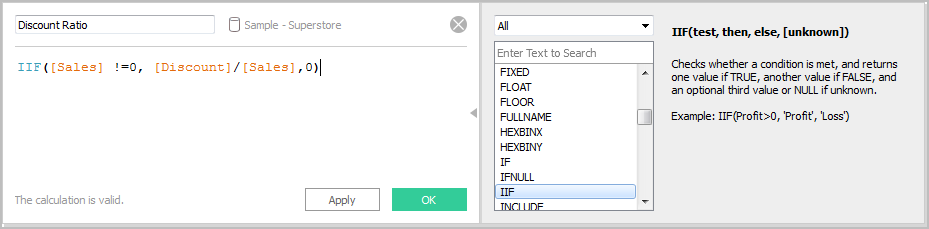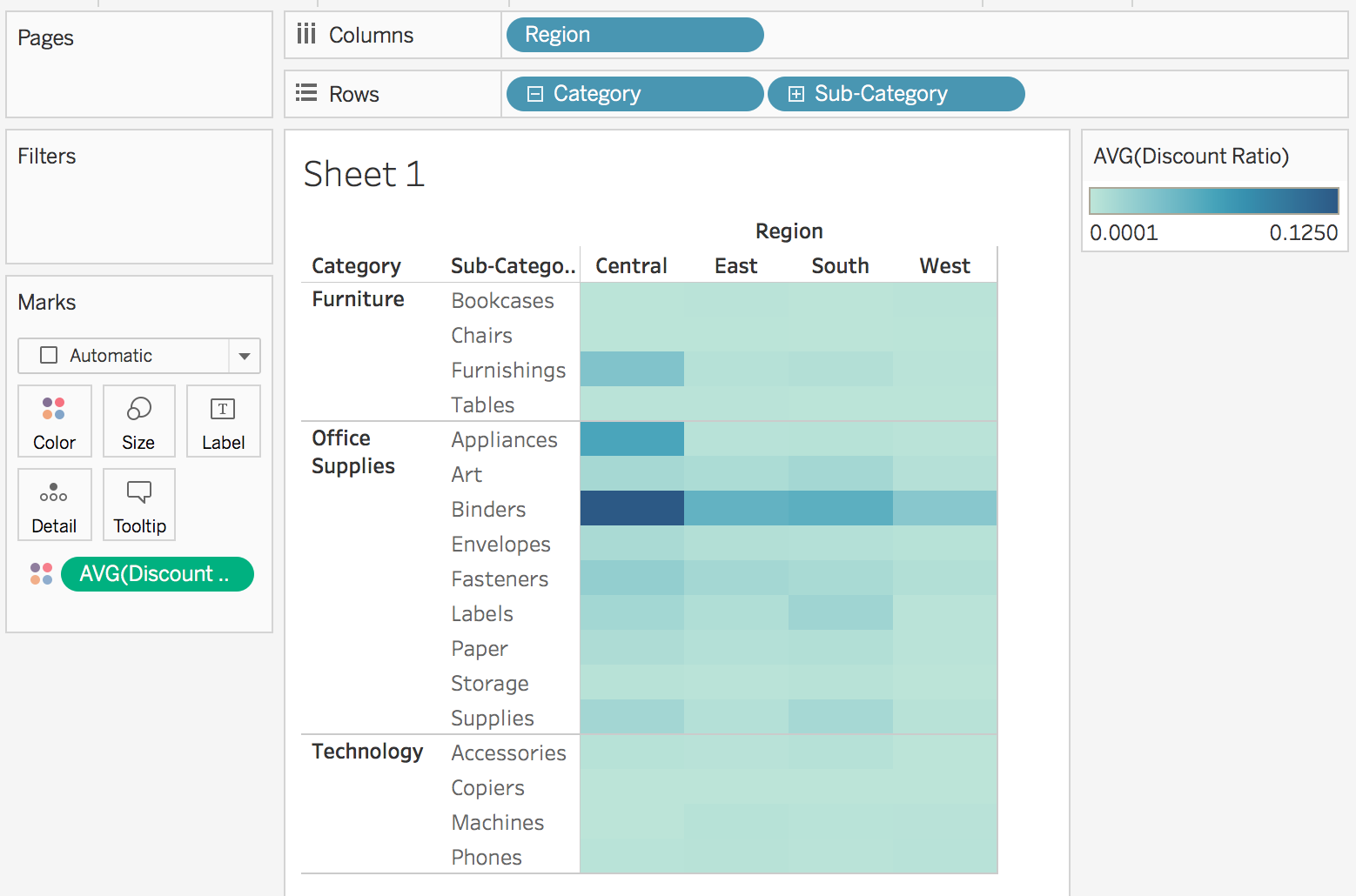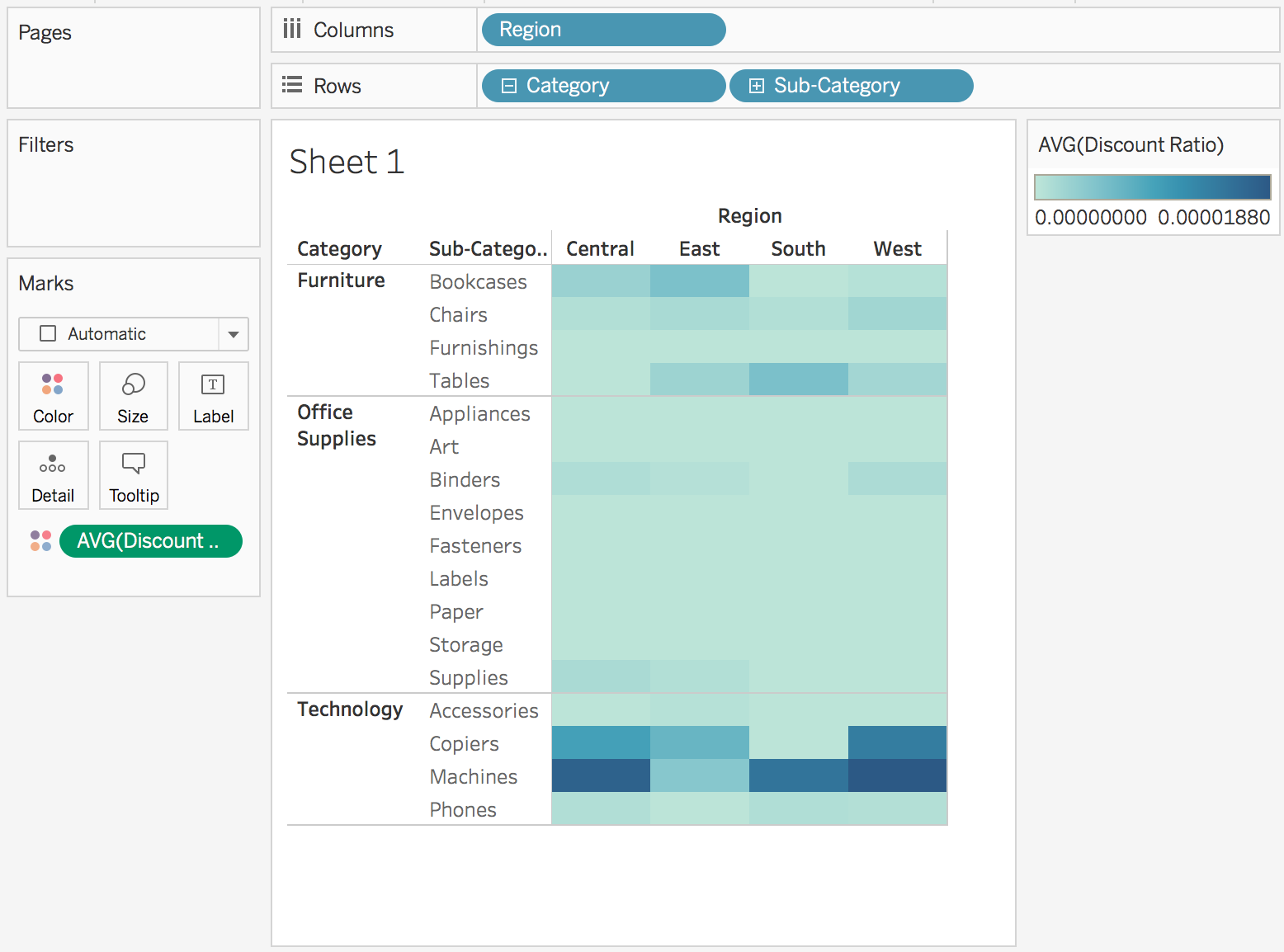This article describes how to create and use calculated fields in Tableau using an example.

You'll learn Tableau calculation concepts, as well as how to create and edit a calculated field. You will also learn how to work with the calculation editor, and use a calculated field in the view.

If you're new to Tableau calculations or to creating calculated fields in Tableau, this is a good place to start.

## Why Use Calculated Fields

Calculated fields allow you to create new data from data that already exists in your data source. When you create a calculated field, you are essentially creating a new field (or column) in your data source, the values or members of which are determined by a calculation that you control. This new calculated field is saved to your data source in Tableau, and can be used to create more robust visualisations. But don't worry: your original data remains untouched.

You can use calculated fields for many, many reasons. Some examples might include:

• To segment data
• To convert the data type of a field, such as converting a string to a date.
• To aggregate data
• To filter results
• To calculate ratios

## Types of calculations

You create calculated fields using calculations. There are three main types of calculations you can use to create calculated fields in Tableau:

• Basic calculations - Basic calculations allow you to transform values or members at the data source level of detail (a row-level calculation) or at the visualisation level of detail (an aggregate calculation).

• Level of Detail (LOD) expressions - Just like basic calculations, LOD calculations allow you to compute values at the data source level and the visualisation level. However, LOD calculations give you even more control on the level of granularity you want to compute. They can be performed at a more granular level (INCLUDE), a less granular level (EXCLUDE), or an entirely independent level (FIXED) with respect to the granularity of the visualisation.

• Table calculations - Table calculations allow you to transform values at the level of detail of the visualisation only. For more information, see Transform Values with Table Calculations(Link opens in a new window).

The type of calculation you choose depends on the needs of your analysis and the question you want to answer.

## Create a calculated field

Once you have determined the type of calculation you want to use, it's time to create a calculated field. This example uses a basic calculation.

Note: The example in this article uses the Sample-Superstore data source that comes with Tableau Desktop. To follow along with the steps in this article, connect to the Sample-Superstore saved data source and navigate to Sheet 1.

1. In Tableau, select Analysis > Create Calculated Field.

2. In the Calculation Editor that opens, do the following:

• Enter a name for the calculated field. In this example, the field is called, Discount Ratio.

• Enter a formula. This example uses the following formula:

`IIF([Sales] !=0, [Discount]/[Sales],0)`

This formula checks if sales is not equal to zero. If true, it returns the discount ratio (Discount/Sales); if false, it returns zero.

 Tip: To see a list of available functions, click the triangle icon on the right-side of the Calculation Editor.Each function includes syntax, a description, and an example for your reference.Double-click a function in the list to add it to the formula.For more tips, see Tips for Working with Calculated Fields in Tableau.
3. When finished, click OK.

The new calculated field is added to Measures in the Data pane because it returns a number. An equal sign (=) appears next to the data type icon. All calculated fields have equal signs (=) next to them in the Data pane.## Use a calculated field in the view

### Step 1: Build the view

1. From Dimensions, drag Region to the Columns shelf.

2. From Dimensions, drag Category to the Rows shelf.

3. On the Rows shelf, click the plus icon (+) on the Category field to drill-down to Subcategory.

The view updates to look like this:### Step 2: Add the calculated field to the view

1. From Measures, drag Discount Ratio to Colour on the Marks card.

The view updates to highlight table.You can see that Binders are heavily discounted in the Central region. Notice that Discount Ratio is automatically aggregated as a sum.

2. On the Rows shelf, right-click SUM(Discount Ratio) and select Measure (Sum) > Average.

The view updates with the average of discount ratio shown.## Edit a Calculated Field

If at any time you need to change a calculation, you can edit the calculated field and it will update across your entire workbook.

To edit a calculated field:

1. In the Data pane, right-click the calculated field and select Edit.

2. In the Calculation Editor that opens, you can do the following:

• Edit the name of the calculated field.

• Update the formula.

For this example, the formula is changed to return a discount ratio for orders over 2000 USD in sales:

`IIF([Sales] > 2000, [Discount]/[Sales],0)`

3. Click OK.

The view updates to reflect the changes automatically. You do not need to re-add the updated calculated field to the view.